## 31.8.05

### Corrupted Maps revisited

Recently, I analyzed the subspace comm range issue a bit. Cabman and AlexD pointed out that subspace comm has less range than the manual and online help suggest. I figured out now that this problem is related to the so called "corrupted maps":

The main map is a grid. Let us assume there are two systems with these coordinates:

System A (Xa|Ya)
System B (Xb|Yb)

(Lord Brazen illustrates here the info of the star table. I used mapleveller to read and alter the save game info for the following examples.)

The distance formula d (A, B) of the F9-Parsec-Display is based on the Euclidean distance, this value is divided by 30 and then rounded up:

(I) d (A, B) = round up [ sqrt { (Xa-Xb)^2 + (Ya-Yb)^2 } / 30 ]

(Rounding up makes sense: When the exact value is 4.5 parsecs we just want the info that we need at least 5 parsecs/turn to cover this distance in one turn.)

The same formula d (A, B) is applied to measure the Subspace Comm Range. The manual states:

This upgrade to all your relay stations gives you the ability to issue orders to any friendly ship within 6 parsecs.

Let us assume system A contains a relay station and B reflects the coordinates of the fleet:
d (A, B) = 5 means then that the fleet is in subspace comm range and
d (A, B) = 6 means that the fleet is out of subspace comm range.

With respect to this function d (A, B) there are now two strange results:

1. Why does it happen that a fleet leaving system A with anti-matter drive (and no navi) is out of subspace comm range the next turn?
2. Why does it happen that a fleet with ion drive (and no navi) sometimes just needs one turn to cover a distance of 5parsecs (according to F9-Info on main map)?

Actually, the answer of these both questions is the same: Rounding. The fleets are also attached to (X/Y)-Positions in the save and each turn a rounding occurs to receive a position on the grid. When you move from System A to B the new coordinates of your fleet are calculated by:

(II) (X|Y) = (Xa|Ya) + round up { (Xb-Xa|Yb-Ya) * c }

The factor c is the rounded travel proportion to receive a intersection on the grid:

(III) c = min { 1; parsec per turn * 30 / round down [ sqrt { (Xa-Xb)^2 + (Ya-Yb)^2 } ] }

(IV) (X|Y) = (Xa|Ya) + round up { (Xb-Xa|Yb-Ya) * min { 1; parsec per turn * 30 / round down [ sqrt { (Xa-Xb)^2 + (Ya-Yb)^2 } ] } }

A few examples:
System A is (always) at position (505|370) now and a fleet with anti-matter drive (5 parsecs per turn) leaves that system to direction B:

a) System B is at (505|520) and we receive then:
(I) d (A, B) = round up  = 5 (is even unrounded exactly 5. System B is exactly below A - same X-Position - and no rounding occurs)
(III) c = 1
and therefore:
(IV) (X|Y) = (505|370) + (0|150) * 1 = (505|520)
The fleet needs 1 turn to move from A to B.

b) Let us change the Y-Position slightly (+1). System B is now at (505|521):
(I) d (A, B) = round up [ 5.03333333] = 6 (6 parsecs is displayed on the main map now - f9)
(III) c = 150/151 = 0.993377
and after 1 turn the fleet is still at:
(IV) (X|Y) = (505|370) + round up [ (0|151) * (150/151) ] = (505|520)
The fleet needs 2 turns to move from A to B.

c) Now same Y-Position like example a) but now a different X-Position (+29). System B is now at (529|520):
(I) d (A, B) = round up [5.06359556] = 6
(III) c = 150/151 = 0.993377
and after 1 turn:
(IV) (X|Y) = (505|370) + round up [ (24|150) * (150/151) ] = (529|520)
The fleet needs just 1 turn to move from A to B. (so called corrupted map because 6 parsecs distance is displayed on main map)

d) Let us change the X-Position a bit further (compared to example c). System B is now at (530|520):
(I) d (A, B) = round up [ 5.06896878] = 6
(III) c = 150/152 = 0.986842
and after 1 turn the fleet is still at:
(IV) (X|Y) = (505|370) + round up [ (25|150) * (150/152) ] = (530|519)
The fleet needs 2 turns to move from A to B.

The SubspaceComm-Issue
Look again at examples b) and d) where the fleet is in hyperspace after the first turn.
In example b) the fleet position (505|520) is exactly 5 parsecs away from system A. And therefore it is in subspace-comm range.
In example d) the euclidean distance is 5.036 parsecs. This value is rounded up to 6 parsecs (see (I)) and the fleet is out of subspace-comm range.
Case b) where no rounding occurs should happen very rarely.

Therefore: A fleet which leaves system A with anti-matter drive (and no navi) is almost always out of subspace comm range the next turn because it is generally a bit faster than 5 parsecs/turn.

Before I figured out the math I thought that the subspace comm issue is just a very late rule change (not mentioned in manual or online help). But it seems now clear that the missing parsec was not intended by the game designers - these rounding issues have been overlooked. Imho it is therefore a bug and should be fixed. There are several options. So far it is only brainstorming, but replacing (IV) with equation (V) looks interesting:

(V) (X|Y) = (Xa|Ya) + round down { (Xb-Xa|Yb-Ya) * min { 1; parsec per turn * 30 / round up [ sqrt { (Xa-Xb)^2 + (Ya-Yb)^2 } ] } }

With this change subspacecomm would work correctly. Also the corrupted maps issue would be affected by this change. It is no longer possible to be faster than expected but slower. (Probably an advantage for the defenders.)

## 12.8.05

### Combat Speed

There is some interesting discussion on LBs forum about the so called battlepods "bug":

To illustrate dirt-bag's proposal look at the combat speed table (click it to enlarge):Combat Speed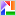These are the EXE-values dirt-bag refers to. You see that the difference between minimum and maximum speed - the maximal unused space speed bonus - is always 10. This table does not include the battlepods effect or augmented engines. Some examples how this bonus works:

I. Status quo

a) destroyer - nuclear drive
the destroyer has 60 space avaiable which we will define as hull size.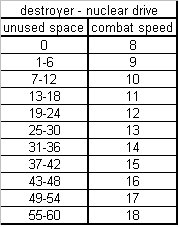DD nuclear driveSo we have:

(I) combat speed = minimum combat speed + unused space bonus

and

(II) unused space bonus = round up [10 * ( unused space / hull size )]

combined

(III) combat speed = minimum combat speed + round up [10 * ( unused space / hull size )]

b) destroyer - nuclear drive - megafluxers
Before we look at the battlepods issue let us see how megafluxers work. Them maximum unused space is now 75. The space increase is abbreviated with dM and it is added to the hull size in (II) and (III). So we have now:

(IV) combat speed = minimum combat speed + round up [10 * ( ( unused space ) / ( hull size + dM) )]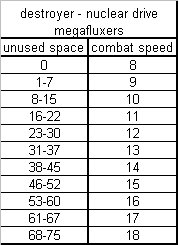DD megafluxers nuclear driveResults:
Maximum combat speed is unchanged. (sounds logical)
Minimum combat speed is unchanged. (counterintuitive)
A DD (which has before and after researching megafluxers the same space consumption) has on average a speed increase of 1.05. (Values vary from 0-2)

c) destroyer - nuclear drive - battlepods
Let us now analyze the battlepods (without megafluxers).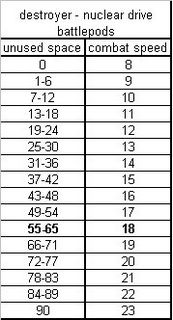DD battlepods nuclear driveFor the first 60 unused space units the equation (II) and the table under b) destroyer - nuclear drive - megafluxers were applied. When there is even more unused space the battlepods bonus comes into play again:

(V) battlepods bonus = round down [10 * dB / hull size ] * round down [ (unused space-60) / dB ]

Results:
Maximum combat speed increases beyond 18. (extremely counterintuitive. That is the main problem we want to solve here.)
Minimum combat speed is unchanged. (counterintuitive)
A DD (same space consumption) has on average a speed increase of 4.59 caused by battlepods. (Values vary between 4-5)

d) destroyer - nuclear drive - battlepods + megafluxers
The results are now a bit incosistent. You cant describe them by "more space=more speed" any longer.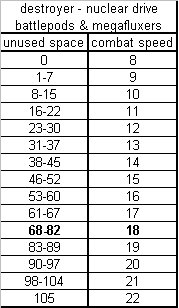DD battlepods & megafluxers nuclear driveFor the first 75 unused space units the equation (IV) and the table under b) destroyer - nuclear drive - megafluxers were applied. When there is even more unused space a further battlepods bonus comes into play:

(VI) battlepods bonus = round down [10 * dB / ( hull size + dM ) ] * round down [ (unused space-75) / dB ]

The megafluxers increase dM is added to the hull size. Therefore some empty battlepods ships even slow down when you research megafluxers.
The maximum combat speed (22) is lower here than under c). (inconsistent)

II. Megafluxers solution for Battlepods
One idea to solve the problem seems straightforward to me. Battlepods should influence the combat speed like megafluxers. Equation (V) is then replaced by

(VII) combat speed = minimum combat speed + round up [10 * ( ( unused space ) / ( hull size + dB ) )]

and instead of (VI) following equation is used:

(VIII) combat speed = minimum combat speed + round up [10 * ( ( unused space ) / ( hull size + dB + dM ) )]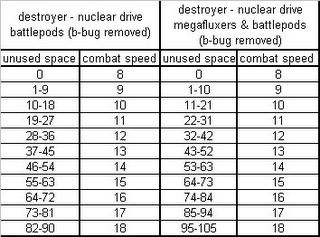Battlepods-"bug" removedResults:
Maximum combat speed stays at 18. (sounds now logical)
Minimum combat speed is unchanged. (still counterintuitive)
A DD (same space consumption) has on average a speed increase of 1.69 caused by battlepods. (Values vary between 0-4)

III. Reducing unused space bonus
Another idea is quite easy to realize and it is one of dirt-bag's preferred solutions. Simply reducing the maximal unused space speed bonus. So far, it was always 10 in the above-mentioned examples. With a maximum of 2 we receive following results (click it to enlarge):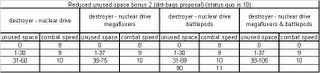Reduced Unused Space BonusResults:
Maximum combat speed stays almost at 10. (battlepods-bug almost completely resolved)
Minimum combat speed is unchanged. (still counterintuitive)
A DD (same space consumption) has on average a speed increase of 0.52 caused by battlepods. (Values vary between 0-1)

Other values than 2 are also an option (especially when combined with Solution II.). A switch which determines the unused space bonus would be a nice option to test different values.

IV. Space Consumption Model

a) Introduction

So far we had formulas which were based on unused space. Such formulas work fine as long as hull size is constant. But with space increases (like megafluxers or battlepods) we had a lot of fuzzy results. As long as unused space is our key variable there is no workaround to solve all the different problems at the same time. But there is a simple space consumption model with imo convincing results. Replace equation (I), (II) and (III) with:

(IX) combat speed = maximum combat speed - space consumption malus

and

(X) space consumption malus = round down [(10/1.5) * ( consumed space / hull size )]

combined

(XI) combat speed = maximum combat speed - round down [(10/1.5) * ( consumed space / hull size )]

The denominator 1.5 achieves that a full battlepod ship will have the same speed as under the status quo. The value reflects the battlepod increase (HS+dB)/HS. This value 1.5 works for dd-doomstar, for a ff the exact value 37/25 should be used.

No further formula is needed. Battlepods or megafluxers don't change the formula - they just increase the potential to consume more space. Therefore we need just one table to display all results (incl. battlepods, megafluxers or battlepods+megafluxers):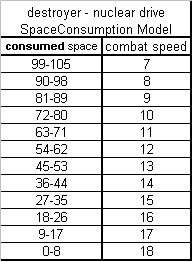Space Consumption ModelResults:
a) an empty destroyer has always combat speed 18. (convincing.)
b) a full destroyer (without bp or m) is now a bit faster than under the status quo (combat speed 12).
c) a full destroyer with battlepods has still the status quo-speed. (8)
d) a full bp-destroyer is therefore slower than a full non-bp-destroyer. (convincing)
e) A DD (same space consumption) has never a speed increase caused by battlepods or megafluxers. (convincing, or at least no completely unconvincing speed changes)

Compare the last point with the megafluxers effect under the status quo: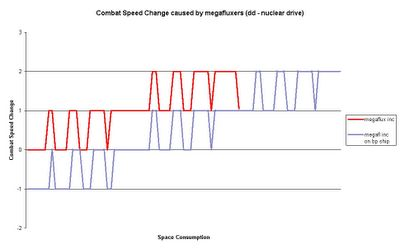megafluxers speed changeLet us assume we have same design and just add megafluxers:
For a non-bp ship see the red graph. (Space consumption 0-60)
BP ship the blue graph. (Space consumption 0-90) There is even the above mentioned speed decrease.

The speed decrease will be eliminated in a pure megafluxers solution (solution II) but results similar to the red graph will persist. And IMO there is no convincing explanation for such behaviour. IMO solutions based on space consumption models (solution IV) should be the way to go.

b) Implementing a battlepods malus

I propose to implement the bp-extra malus (dirt bag's idea here) in the following way:

Just change the factor (10/1.5) to 9/1.5=6
(Once again, for a ff the exact value 37/25 should be used.)

So instead of (XI) we have:

(XII) combat speed = maximum combat speed - round down [6 * ( consumed space / hull size )] and -1 when bps are used.SC Model with BP-Malus61-75 depends on battlepods.
0-60 using battlepods is inefficient.
76-105 using them is necessary.

Adding BPs and consuming the extra space is always a loss of 4 combatspeed and -20 beamdefence then.

c) Implementing an unused space bonus reduction

Dirt-bag's observation is correct that this new model doesn't solve the runner-problem completely. But there is a simple way to implement such idea (solution III), which just reduces the maximum combat speed (and not the speed of a full battlepod ship):

(XIII) combat speed = maximum combat speed - C - round down [(9-C)/1.5 * ( consumed space / hull size )] and -1 when bps are used.

(Once again, for a ff the exact value 37/25 should be used.)

The best example to illustrate the runner problem is IMHO a ff on iondrive level. In the following table (click it to enlarge) you see the results for different C-Values (0-9):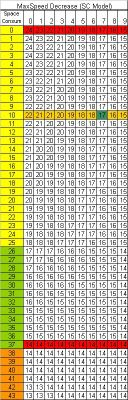SpeedDecreaseSCModelIn the red row at top you see the maximum speed decrease. The lower red row shows that the speed for a full bp-ff is unchanged. The beige row is important for aug eng ffs. (aug eng consumes 10 space). Just add 5 combat speed to this row and you receive the combat speed of these runners.

According to dirt-bag a runner with 22 combat speed is unable to outrun a fast nuke (on iondrive-lvl 18 speed). The C-Value of 7 should be his preferred solution then.